##全国校区# 快速解释如何使用pandas的inplace参数2020-07-22

pandas 是源于NumPy 的一种python库，主要是为了解决数据分析任务而创建的。pandas为我们提供了大量简单便捷地处理数据的函数和方法。今天小编给大家分享的就是：快速解释如何使用pandas的inplace参数，希望对大家学习和使用pandas有所帮助。

# 介绍

fillna()dropna()sort_values()reset_index()sort_index()rename()

# 创建一个示例DataFrame

``` import pandas as pd
import numpy as np
client_dictionary = {'name': ['Michael', 'Ana', 'Sean', 'Carl', 'Bob'],
'second name': [None, 'Angel', 'Ben', 'Frank', 'Daniel'],
'birth place': ['New York', 'New York', 'Los Angeles', 'New York', 'New York'],
'age': [10, 35, 56, None, 28],
'number of children': [0, None, 2, 1, 1]}
df = pd.DataFrame(client_dictionary)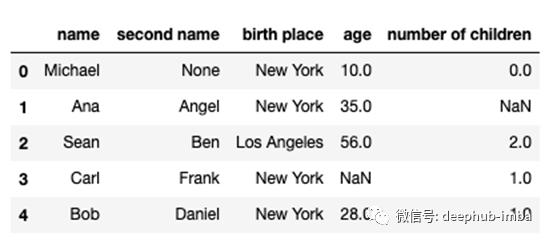``` df_1 = df.copy()
df_2 = df.copy()```

` df_1.dropna(inplace=True)`

` df_1.head()`

` df_2.dropna(inplace=False)`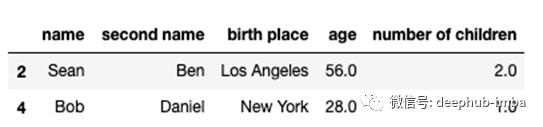` df_2.head()`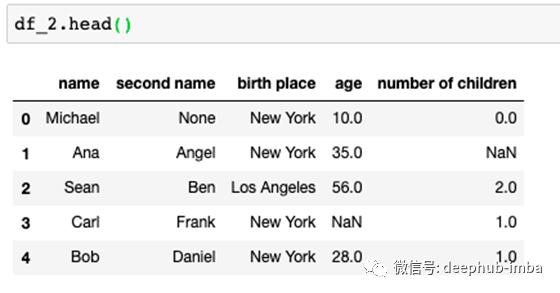` df_2 = df_2.dropna(inplace=False)`

` df_2.dropna(inplace=True)`

# 常见错误

` df[['second name', 'age']].dropna(inplace=True)`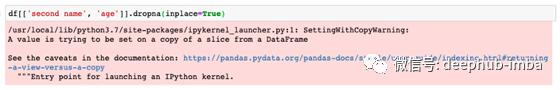``` df.dropna(inplace=True, subset=['second name', 'age'])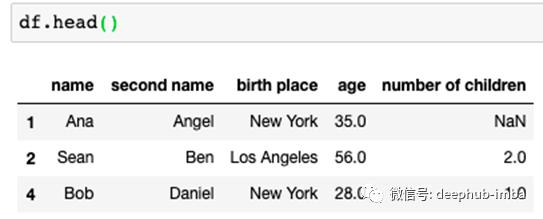` df = df.dropna(inplace=True)`

# 总结

OK